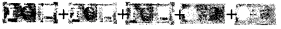# AP Board 3rd Class Maths Solutions 2nd Lesson Numbers

Andhra Pradesh AP Board 3rd Class Maths Solutions 2nd Lesson Numbers Textbook Exercise Questions and Answers.

## AP State Syllabus 3rd Class Maths Solutions Chapter 2 Numbers

Textbook Page No. 12

I. Observe the following picture:

Bindu went to a household exhibition cum sales center with her mother. They wanted to buy the following items. Observe the items and their price tags. Bindu started to read the cost of these items, please help her.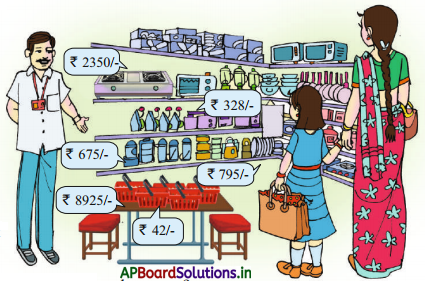Question 1.
What are the items do you observe in this sales center?
The items are hot box, vegetable basket, tiffen box, gas stove, mixers, ovens, pans, fans etc.

Question 2.
What is the price of hot box?
The price of hot box is ₹ 795

Question 3.
What is the price of thermos flask?
The price of thermos flask is ₹ 675Question 4.
What is the price of vegetable basket?
The price of vegetable basket is ₹ 42

Question 5.
What is the price of gas stove?
The price of gas stove is ₹ 235

Do these

Write the numbers in words.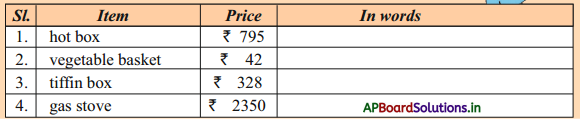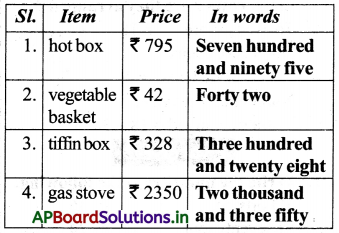Textbook Page No. 15

II. The numbers after 1000:

Which is the next number to 1000?
1000 + 1 = 1001

Which is the next number to 1001?
1001 + 1 = 1002

Like wise, let us prepare a chart of numbers that come after 1000 and read.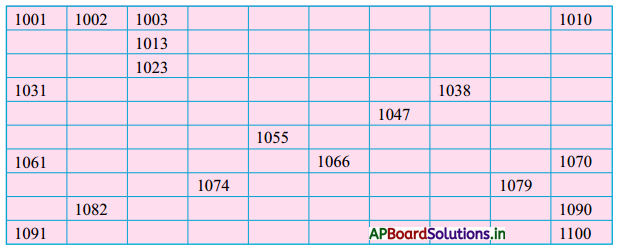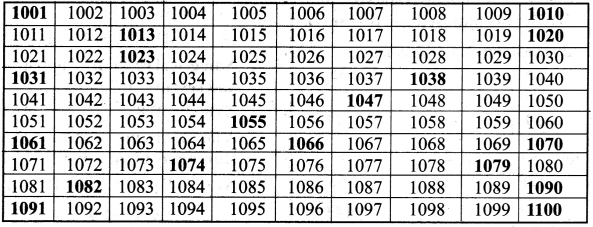Textbook Page No. 16

Do these:

Question 1.
Write the correct digit in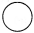and write the number in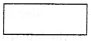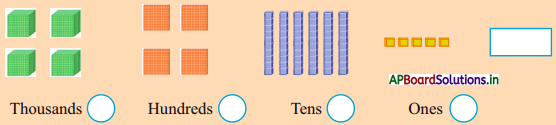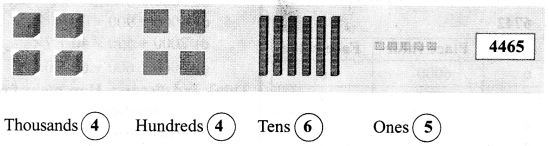Textbook Page No. 17

Question 2.
Write the number and number name by observing beads on the abacus. One is done for you.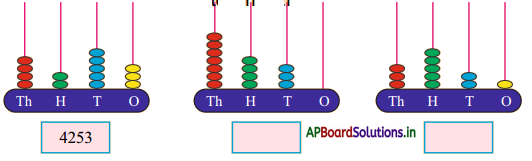a) Four thousand two hundred and fifty three
b) ____________________
c) ___________________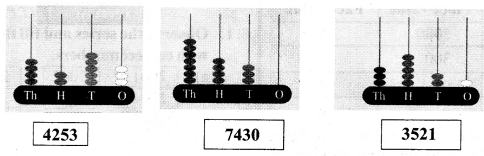a) Four thousand two hundred and fifty three
b) Seven thousand four hundred and thirty
c) Three thousand five hundred and twenty oneQuestion 3.
Mr. Pradeep has to write a cheque for 3,456. Help him to write the amount in words.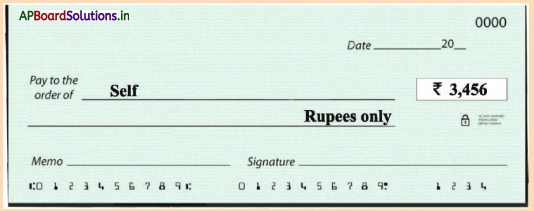Three thousand Four Hundred and Fifty Six.

Textbook Page No. 18

III. What did you notice above?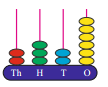Which digit is in the ones place? 6 it’s place value is 6.

Question 1.
Which digit is in the tens place? __________ , it’s place value is _____.
2, 20

Question 2.
Which digit is in the hundreds place? _____, it’s place value is ____.
3, 300Question 3.
Which digit is in the thousands place? _____, it’s place value is _____.
2, 2000

Do these:

1. Find that place value and face value of each of the digits in the following numbers.

a) 6742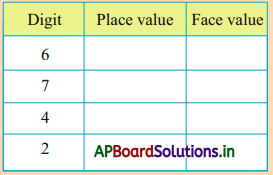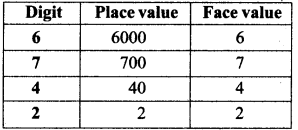b) 5309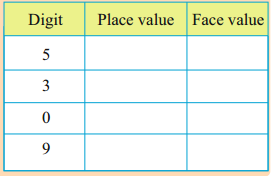Textbook Page No. 19

Question 2.
Encircle the place value of the digits underlined in the give numbers ?
One is done for you.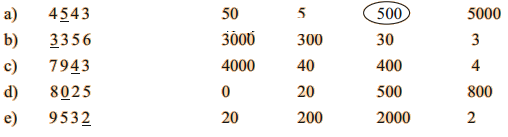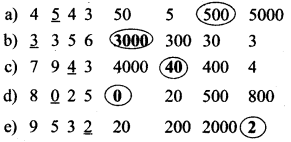Question 3.
For each of the number given below, match the place value and face value of the circled digits by drawing line as shown below.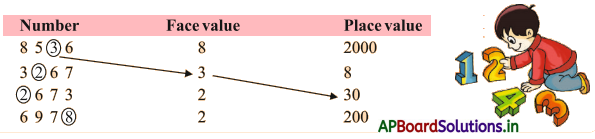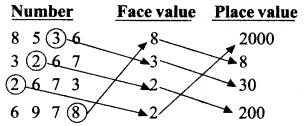Do these :

1. Write the expansion form the following numbers.

a) 4354 = ______
4000 + 300 + 50 + 4

b) 4199 = __________
4000 + 100 + 90 + 9c) 7575 = ______
7000 + 500 + 70 + 5

d) 6402 = _____
6000 + 400 + 00 + 2

Textbook Page No. 20

2. Match the following expansion form with their short form.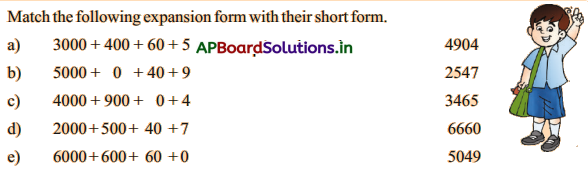Think and discuss:- How many four digit numbers are there in all?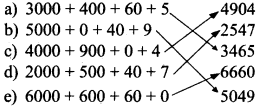The smallest 4 digit numbers = 1000
The greatest 4 digit numbers = 9999
Total 4 digit numbers = 9999 – 1000 + 1 = 9000

Exercise 1

1. Observe the series and fill the boxes with correct numbers.
a)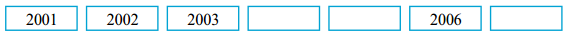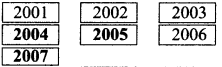b)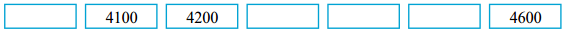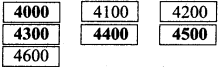2. Read the numbers given in words below and write in numbers (Numerals) in the boxes.

a) Three thousand five hundred and twenty five = ____
3525

b) Seven thousand seven hundred and eight = _____
7708

c) Eight thousand and five = _____
8005

3. Write the place value of encircled digits.

a)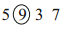________
900

b)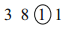= ______
10

c)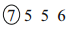= _____
7000d)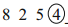= _____
4

4. Write the following numbers in words.

a) 5876 – ___________________
Five thousand eight hundred and seventy six

b) 7305 – ________
Seven thousand three hundred and five.

c) 4975 – ________
Four thousand nine hundred and seventy

d) 2089 – ________
Two thousand and eighty nine

5. Write each of the following in expanded form:

a) 3870 = _____ + ____ + _____ + _____
3000 + 800 + 70 + 0

b) 7077 = ____ + _____ + ____ + ____
7000 + 000 + 70 + 7

c) 9330 = ____ + ____ + _____ + _____
9000 + 300 + 30 + 0

Textbook Page No. 21

6. Fill in the blanks with the missing place value.

a) 5000 + ____ + 90 + 3 = 5693
600

b) ____ + 600 + 0 + 5 = 3605
3000

c) 6000 + ____ + 70 + 7 = 6177
100

d) 9000 + 900 + ____ + 9 = 9999
90

7. Write the following in shortened form in numerals ?

a) Five Thousand + Two Hundreds + Forty + Three = _____
5243

b) Seven Thousand + One Hundred + Sixty+Eight = ____
7168

c) One Thousand + One Hundred + One = ______
1101

d) Two Thousand + Thirty + Five = _____
2035Question 8.
Write the four digit number having 5 in thousands place, 8 in hundreds place, 3 in tens place and 2 in ones place.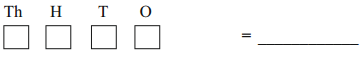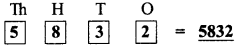Question 9.
Write the 4-digit number having 2 in ones place, 5 in tens place, 0 in hundreds place and 6 in thousands place.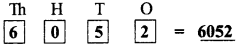Activity :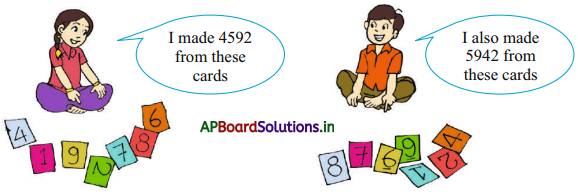• Take any 4 cards from 0 to 9.
• Make any 4-digit number with those cards.
• Read and write the number in words.
• Write as many as numbers you can form with those digits in the following table.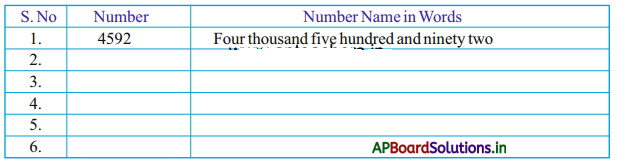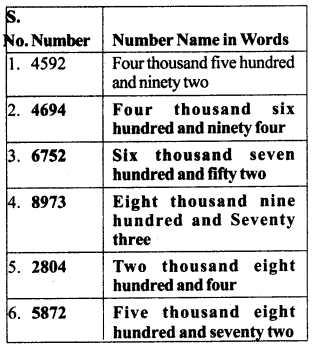Textbook Page No. 22

Try these :

Question 1.
Write all possible 2- digit numbers using the digits 3, 5 and 1.
35, 53, 51, 15, 13, 31

Question 2.
Write any five 4 digit numbers using the digits 2, 6, 8 and 4.
2684, 6842, 8426, 4268, 2864, 6482

Question 3.
If A = 0, B = 1, C = 2, D = 3, E = 4, then find out the price of objects given below using secret code. One is done for you.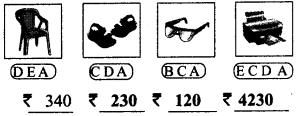Textbook Page No. 24

Do these :

1. Fill in the boxes with < or > symbols.
a)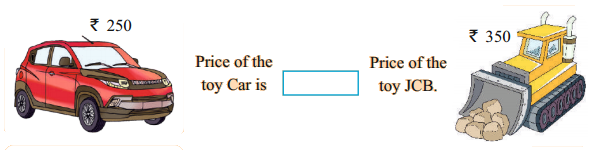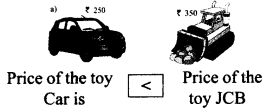b)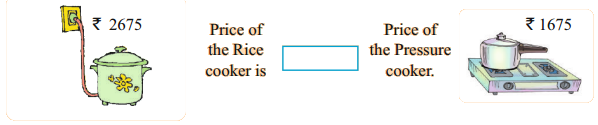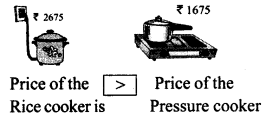2. Write the correct symbol (<, = or >) in the underline given below.

a) 6472 ____ 5306
6472 > 5306

b) 465 ____ 3079
465 < 3079

c) 5780 ____ 5967
5780 < 5967

d) 6504 ____ 6079
6504 > 6079

e) 3281 ___ 3896
3281 < 3896

f) 4650 ___ 4698
4650 < 4698

g) 7856 ____ 7854
7856 > 7854

h) 6702 ____ 6923
6702 < 6923

i) 5063 ____ 5063
5063 < 5063

j) 5716 ____ 5186
5716 > 5186

Textbook Page No. 25

3. Encircle the smallest number in the following. One is done for you.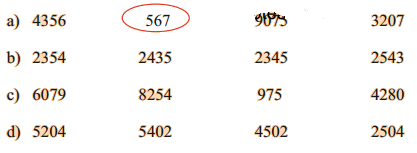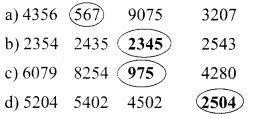Question 4.
Put a (✓) mark to the largest number in the following.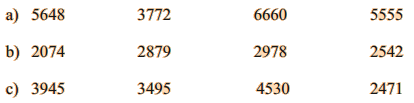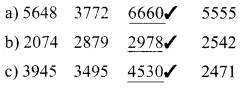IV. Ordering of numbers: Observe these pictures of investment details of four merchants and answer the following questions.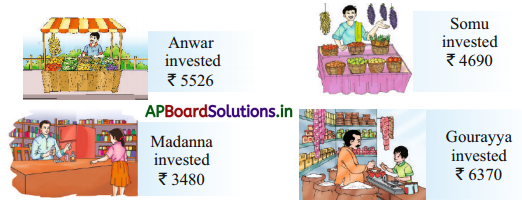a) Who invested more money ? _____ How much ? ______
Gourayya, ₹ 6370

b) Who invested less money ? ____ How much ? _____

c) Write the names of the merchants according to their investments from less to more.
______, ______, _____, ______

d) Write the investments of the merchant from less to more.
______, ______, _____, ______

Textbook Page No. 26

Do these :

Question 1.
Arrange the following numbers in ascending order.
72, 27, 16, 108, 61
Ascending order _____, _____, _____, _____, _____
16, 27, 61, 72, 108Question 2.
Arrange the following numbers in descending order.
65, 506, 650, 560, 605.
Descending order ___, _____, _____, _____, ______
650, 605, 560, 506, 65

Question 3.
1009, 4002, 6088,3800.
Write the numbers by observing the symbols.
a) ___ > _____ > _____ > _____
6088 > 4002 > 3800 > 1009

b) _____ < _____ < ____ < _____
1009 < 3800 < 4002 < 6088

Question 4.
Arrange the following numbers both in ascending and descending order.
2566, 2988, 2300, 2377
Ascending order: _____, ____, ___, _____, _____
2300, 2377, 2566, 2988

Descending order: _____, ____, ___, _____, _____
2988, 2566, 2377, 2300

Textbook Page No. 27

Excercise – 2

Question 1.
Observe and fill the number chart. Write all the numbers having ‘3’ in tens place in the given blanks.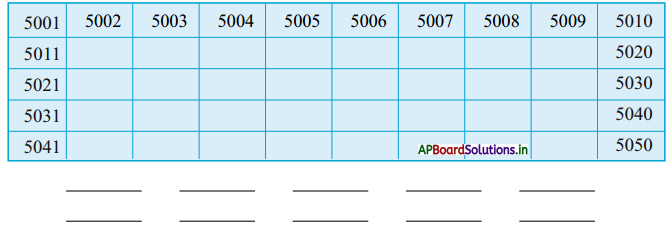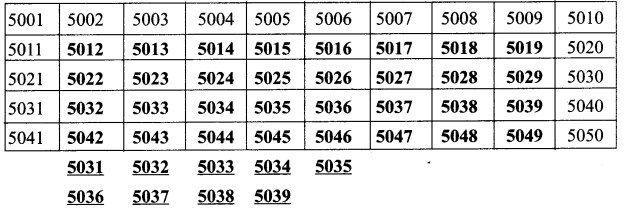Question 2.
Sumathi has the following currency notes. How much money does Sumathi have ?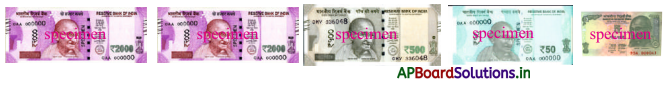₹ 2000 + ₹ 2000 + ₹ 500 + ₹ 50 + ₹ 5
= ₹ 4555

Question 3.
Write the numerals for each of the following:
a) Seven thousand and seventy seven: ______
7077

b) Eight thousand nine hundred and sixty seven: ________
8967

Question 4.
Write the number name for each of the following.
a) 3003 = ______
Three Thousand three

b) 6010 = ______
Six thousand ten

c) 9909 = ______
Nine thousand and Nine hundred and nine

Question 5.
In number 2768,2 is in which place? []
a) Ones
b) Ten
c) Hundreds
d) Thousands
d) Thousands

6. Write the expansion form of the following numbers.
a) 5004 = _______
Five thousand and four

b) 2069 = _______
Two thousand and sixty ninec) 3678 = ______
Three thousand and six hundred and seventy eight

Textbook Page No. 28

Question 7.
Observe the number chart and fill in the blanks. (Some may have multiple answers)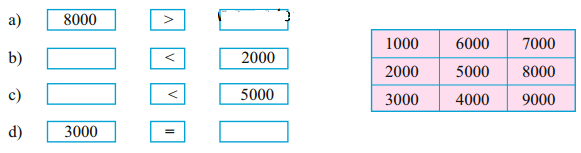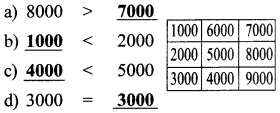Question 8.
In each of the following circle the large number.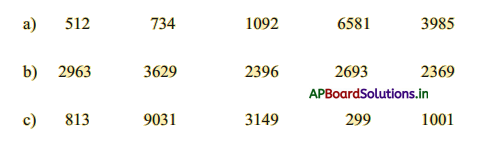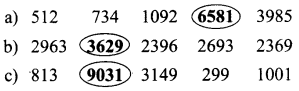Question 9.
Write any six 4-digit numbers using the digits 2, 5, 7 and 8. Then find out the largest and smallest number from them.
a) ______
b) _______
c) ________
d) _______
e) _______
f) _______
a) 8752 (Largest)
b) 5827
c) 8572
d) 5728
e) 7825
f) 2578 (Smallest)

Question 10.
How many different 4-digit number can you arrange using the digits 1, 1, 9 and 9 Write them ?
Four, these are
1199, 1919, 1991, 9911

Question 11.
Some numbers are given below. Circle the range that the number lies in.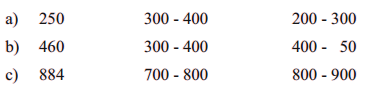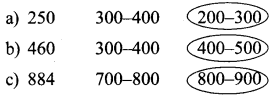Question 12.
Observe the population of the villages and circle to the nearest thousands.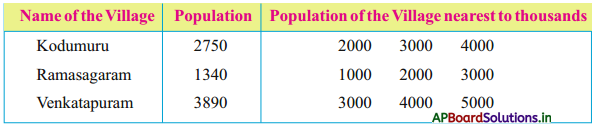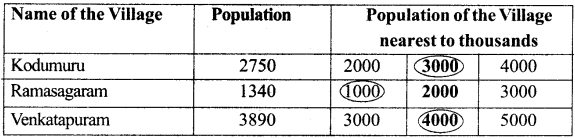Textbook Page No. 29

Question 13.
Circle the nearest number and write it in the given blank. One is done for you.
a)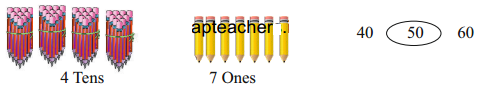The number of pencils are nearer to 50

b)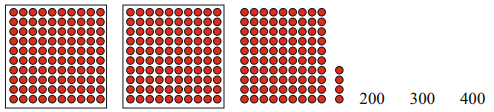The total currency is nearer to ………..
3000

c)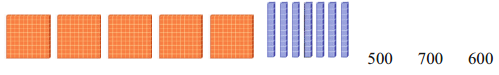The number of blocks are nearer to ……..
600d)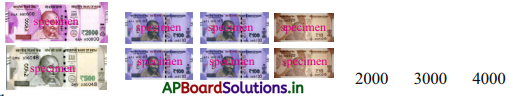The total currency is nearer to ……….
3000

Question 14.
Write the largest and smallest numbers formed by the given digits.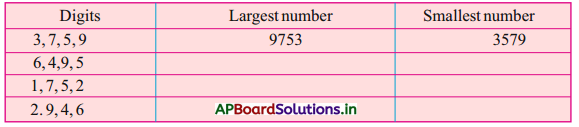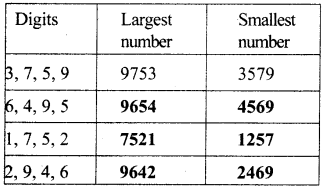Project work: Student Activity:

Collect vehicle numbers and write it in the table.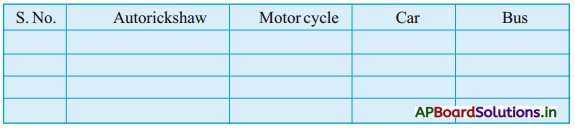a) Write the numbers of Autorickshaws in ascending order.
b) Write the numbers of motor cycles in descending order.

Multiple Choice Questions

Question 1.
Short form of 3000 + 400 + 50 + 6 ()
A) 3450
B) 3560
C) 3456
D) 3546
C) 3456

Question 2.
Among the following which is greater one ? ( )
A) 5476
B) 6123
C) 2689
D) 6542
D) 6542

Question 4.
Expansion form of2377 ( )
A. 2000 + 30 + 70 + 00
B. 2000 + 300 + 70 + 7
C. 2000 + 70 + 300 + 00
D. 20 + 700 + 3000 + 7